# Algebra I Assignment - Arithmetic, Square Roots, Absolute Value & Matrices

Instructor: John Hamilton

John has tutored algebra and SAT Prep and has a B.A. degree with a major in psychology and a minor in mathematics from Christopher Newport University.

This end-of-year math homeschool assignment covers some fundamental Algebra I concepts, including basic arithmetic, square roots, absolute values, and matrices. The review assignment is designed to assist the 9th grade Algebra student. Updated: 03/03/2021

## Assignment Explanation and Topic Overview

Arithmetic. Square roots. Absolute value. Matrices. These are commonly known as the four food groups, and . . . never mind, those are my notes for a nutrition lesson. These are commonly known as four Algebra I concepts which will help you when you move on to more advanced mathematical concepts.

In addition to what is mentioned above, this synoptic assignment will cover matrices and order of operations.

By the end of this assignment, your student(s) will have completed four steps, solved five problems, and delivered two presentations.

Note - The answers are available at the bottom of the page.

## Key Terms

• Absolute value: the size or magnitude of a number disregarding the sign
• Arithmetic: a branch of math which deals with manipulating numbers via four methodologies
• Matrices: rectangular arrays of numbers used to perform math operations

### Materials

• Internet access
• Paper
• Pencil

### Time / Length

• One day to complete this Algebra I material
• Two weeks to design two appropriate presentations

## Assignment Instruction for Students

### Step One - Basic Math Concepts

Before we move on to our review of more advanced Algebra I concepts, it is always helpful to complete a review of some of our basic arithmetic concepts.

First, do you remember the four arithmetic operations, which are addition, subtraction, multiplication, and division?

Second, the commutative property states the following:

3 + 1 = 1 + 3

Third, the associative property states the following:

(5 + 9) + 2 = 5 + (9 + 2)

Fourth, the identity property states the following:

8 + 0 = 8

One other fact we should note is that subtraction is considered the opposite, or the inverse, of addition, and division is considered the opposite, or the inverse, of multiplication.

A number line is utilized to visually represent real numbers. Our number system is a base-ten system, as opposed to say the base-two system used by computers.

By the way, don't forget it is crucial you follow your order of operations, or in order:

1. Parentheses
2. Exponents
3. All multiplication and division from left to right
4. All addition and subtraction from left to right

Hmmm! If we only had some sort of memory trick to help us with this rule. I think I'll invent one right now, and call it PEMDAS, or something similar.

PEMDAS = Parentheses, Exponents, Multiplication, Division, Addition, and Subtraction

Let's work a problem.

#### Problem #1

Use PEMDAS to solve:

8 + (19 - 7 * 2) - 3 * 5

### Step Two - Square Roots

To simplify square roots, it is nice to turn to our product rule, which is:

• √(ab) = √(a) * √(b)

or

• (ab)1/2 = a1/2 * b1/2

This reads as "the square root of quantity a times b equals the square root of a times the square root of b."

By the way, for this formula a and b are not negative numbers.

Simplify:

√150

### Step Three - Absolute Value

Now let's review the concept of absolute value, which is pretty straightforward. For example:

#### Problem #3

Find the absolute values of 5 and -5: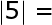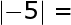#### Problem #4

Find the absolute value of: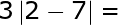## Step Four - Matrices

Next, let's recap the concept of matrices, which are grids of given numbers that we divide into rows and columns. We then place the numbers in brackets for the purpose of calculations.

#### Problem #5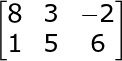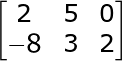## Deliverable

At this point, please choose two of the following presentations:

1. Create questions for a math quiz show based on one of your favorite game shows. Try doing an internet search for free game templates to set up your own game to play with others (suggested search terms: free Jeopardy template).
2. Design a synoptic poster that contains the year-end information in this lesson, and use this poster to answer a series of questions related to the math material.
3. Go ahead and ''be the teacher'' and walk through what you learned throughout this school year in your own TED Talk or YouTube video.
4. Write thoughtful questions to assess understanding of these Algebra I concepts and processes.

#### Solution #1

Use PEMDAS to solve:

8 + (19 - 7 * 2) - 3 * 5 =

To unlock this lesson you must be a Study.com Member.

### Register to view this lesson

Are you a student or a teacher?